# iCE Bling FPGA – Beautiful LED Earrings with Lattice iCE40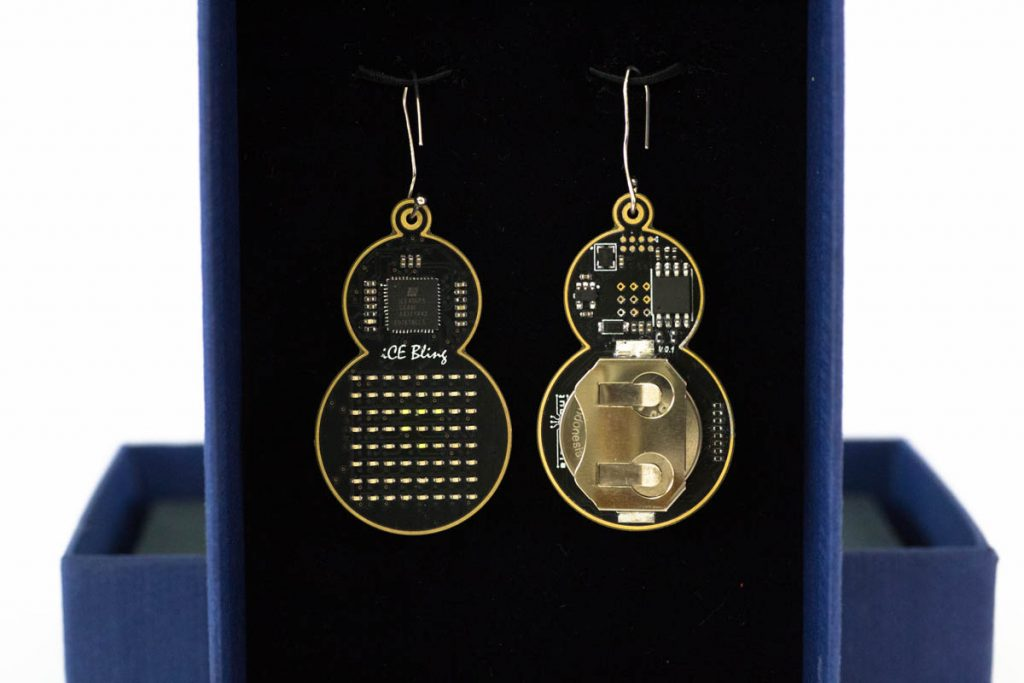It’s the same story every year. At the horizon is a loved one’s birthday, or an anniversary, and I want to make them something special. Buying something won’t do. Oh no, I have to design and build it myself. I would then start with a simple idea, and then complicate it progressively to the point where it would take several anniversaries to finish the project.

This time, I wanted to build a pair of earrings for my wife’s birthday. Since I am learning about FPGAs these days, I wanted to incorporate one into the design. Having gotten older and wiser, I decided to enlist help early on. I would focus on the overall design and the programming part, and leave the PCB design and assembly to my trusted friend and engineer Siva.

### Objective

Build a pair of LED earrings using the Lattice iCE40UP5k FPGA. The earrings would have an 8 x 8 grid of LEDs, and would be powered by a CR2032 coin cell.

### Design Notes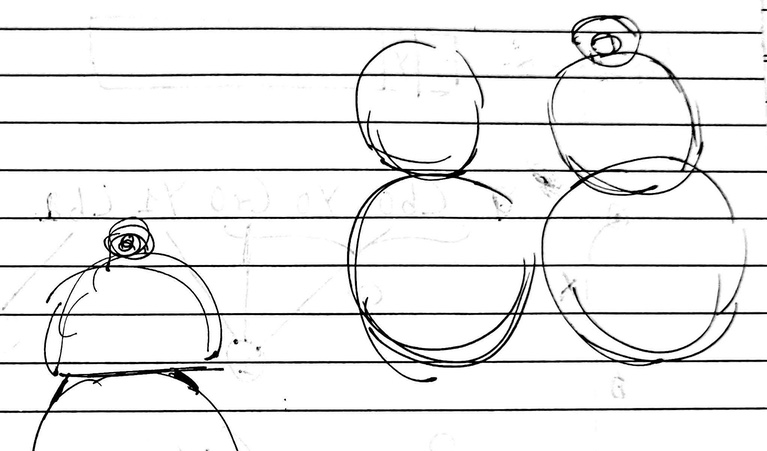My wife likes to wear big earrings, so using a CR2032 was not a problem. The earrings needed to balance nicely, so the battery had to be at the bottom. After considering many crazy shapes, I settled on basic geometry – three circles intersecting slightly.

The 64 LEDs (0402 package) would be arranged in a square grid of 8 x 8. To keep things simple, and since we had a space constraint, I decided not to use shift registers. I would drive the LEDs directly with the FPGA pins, which meant that I had restrictions on how many LEDs could be lit at a given instance. (Due to the current limit on the FPGA GPIO pins.)

Choosing green LEDs had two advantages. It matched my wife’s birthstone colour, and the lower turn-on voltage meant I could safely use the 3V coin cell.

The PCB needs to have a quick way to upload the code, and for that we decided on a 2 x 5, 1.27 mm pitch SMD header. More on that later.

### Prototyping with Lattice iCE40 FPGA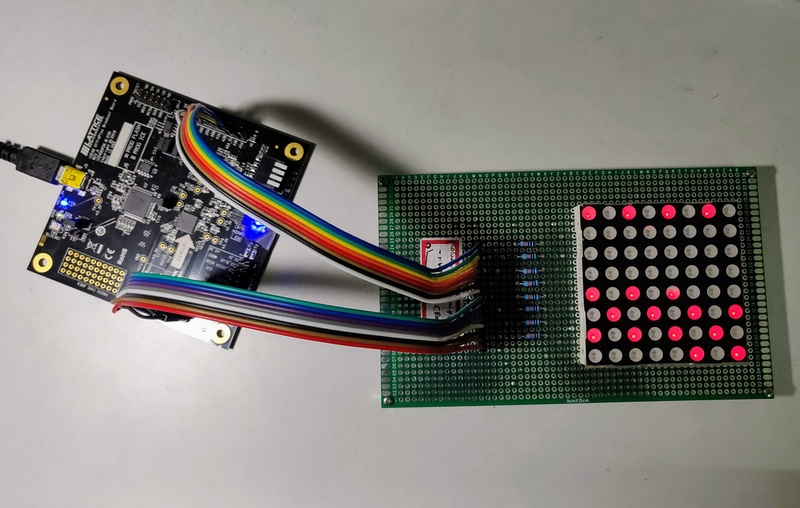I knew that developing and testing code directly on the iCE Bling PCB would be painful, so I used an 8 x 8 LED dot grid display to speed up development. Siva was kind enough to make an adapter PCB for me.

### Schematic

Here’s the schematic for iCE Bling. (PDF available at the download link at the end of this article.)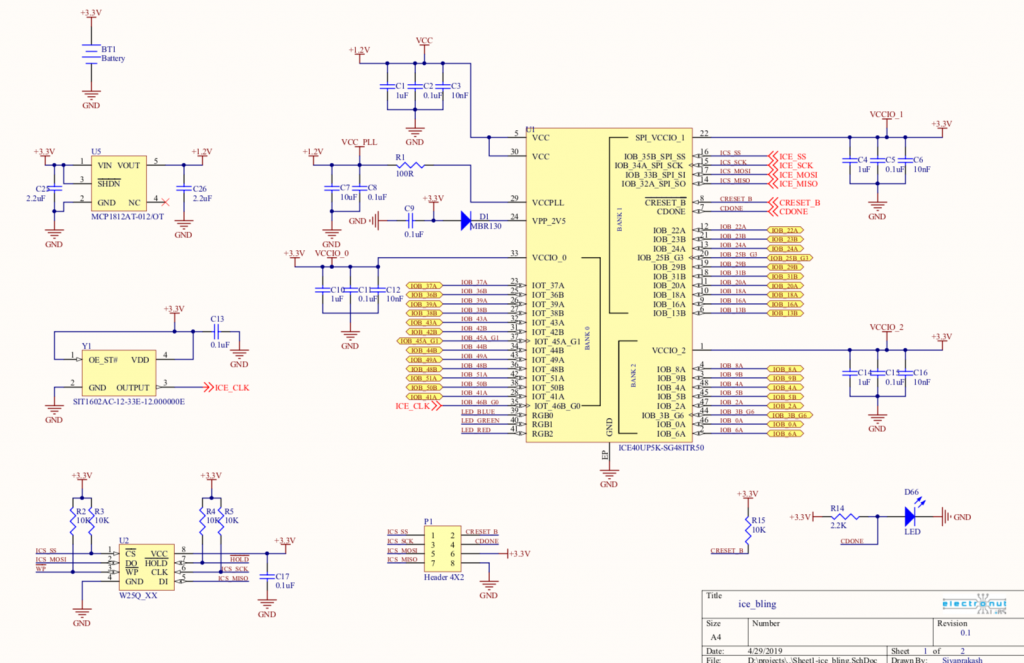So as you can see, not too many components. Just what’s necessary to supply voltages for the FPGA, an SPI flash chip to store the bitstream (program), and an oscillator for the clock. The LEDs are directly connected to the FPGA pins, as I mentioned earlier.

### PCB Design

Here’s what the PCB looks like, in Altium Designer. We had to use a 4-layer design due to restrictions on size and the considerable number of lines that need to be routed.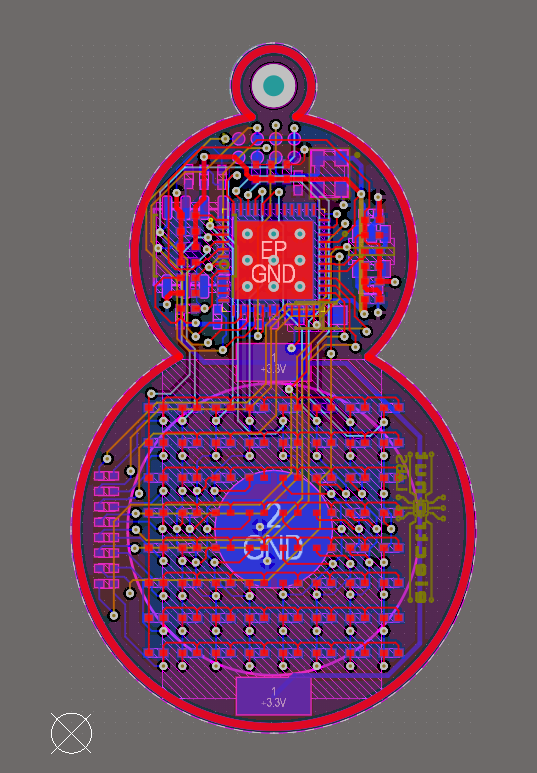As you can see above, the design uses classic shapes, with a hole for a steel hook, and there’s also a gold trace than runs along the boundary, which I think goes well with a black PCB.

### Programmer PCB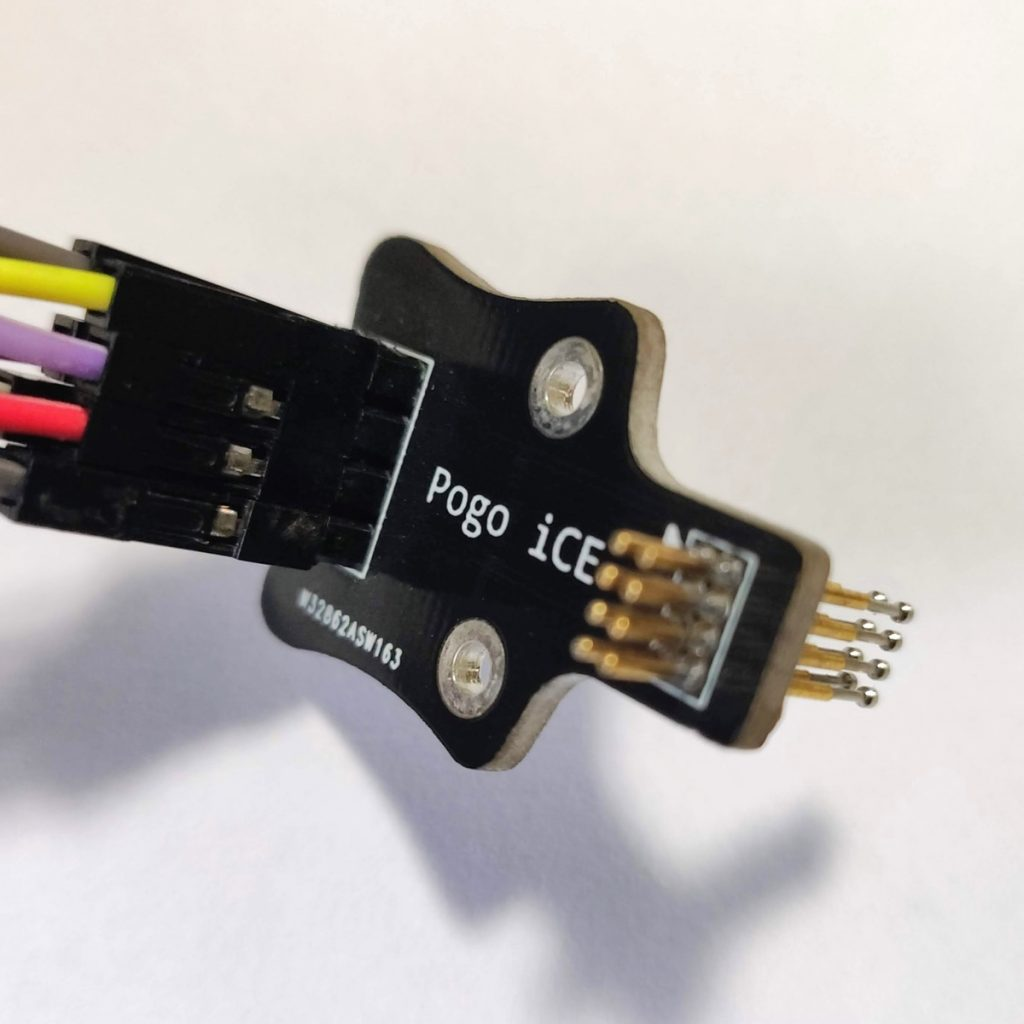I wanted to make it easy to program iCE Bling, so we made special Pogo Pin adapter for it, similar to PogoProg sold by Electronut Labs. It’s designed to be used with an FT232H board, which we will look at further down in the article.

### Logic Design

I used Verilog HDL for this project. Let’s look at the various pieces of logic needed to achieve our goal.

#### Frame Buffer

Since I did not use a shift register, and the FPGA has a current limit of less than 8 mA per GPIO pin, the strategy used was to go through the grid and pulse every LED for a short period of time.

The 8 x 8 grid is represented as a Verilog array reg [63:0] fb. Here are the highlights from the frame buffer display logic in dot88.v.

  always @ (posedge clk)
begin

// initialise
if (!resetn)
begin
pcounter <= 0;
d88_counter <= 0;
xp <= 0;
yp <= 0;
end

d88_counter <= d88_counter + 1;

if (!d88_counter)
begin
pcounter <= pcounter + 1;

// calculate position
xp <= pcounter/8;
yp <= pcounter - 8*(pcounter/8);

// update row/col
c <= fb[pcounter-1] ? (8'd1 << (7 - xp)) : 8'd0;
r <= ~(8'd1 << (7 - yp));
end

end

assign {row, col} = {r, c};


In the above code, all we’re doing is turning on the correct LEDs very fast. We do that by incrementing a mod-64 counter, and setting the correct bits in the row (r) and column (c) buses. If you increase the bit width of d88_counter, you can observe how the display is working.

Now that we know how to display an 8 x 8 “frame” of 64 bits, let’s create some content for it.

#### Scrolling Letters

The first thing I wanted was to scroll the initials of my wife’s name. For this, we define two “frame buffers” and switch between them. The code for this module is in letters88.v.

// "H"
r_fb1[0:7]   = 8'b01000010;
r_fb1[8:15]  = 8'b01000010;
r_fb1[16:23] = 8'b01000010;
r_fb1[24:31] = 8'b01111110;
r_fb1[32:39] = 8'b01111110;
r_fb1[40:47] = 8'b01000010;
r_fb1[48:55] = 8'b01000010;
r_fb1[56:63] = 8'b01000010;


The above code shows how the letter “H” is stored.

// scroll letter 1
r_fb1[0:7]   <= {r_fb1[6:0],  r_fb1};
r_fb1[8:15]  <= {r_fb1[14:8], r_fb1};
r_fb1[16:23] <= {r_fb1[22:16],r_fb1};
r_fb1[24:31] <= {r_fb1[30:24], r_fb1};
r_fb1[32:39] <= {r_fb1[38:32], r_fb1};
r_fb1[40:47] <= {r_fb1[46:40], r_fb1};
r_fb1[48:55] <= {r_fb1[54:48], r_fb1};
r_fb1[56:63] <= {r_fb1[62:56], r_fb1};


The above code shows how the scrolling is done on a clock pulse. Each line is just rotated by one bit.

Now let’s look at the next frame buffer animation.

#### Conway’s Game of Life

I’ve had a fascination for Conway’s Game of Life (GOL) for a while now. It appears in my book Python Playground, as well as some of my blog articles on this website. Not that I pretend to understand what’s going on in GOL – darn thing has a Universal Turing Machine inside of it! Anyway, give me an X/Y grid of anything, and I am likely to try and run GOL on it. The code for this module is in conway88.v.

Here are the rules for GOL on a discrete grid, assuming a value of 1 means “ON” and 0 means “OFF”.

1. Compute 8 nearest neighbour sum of a pixel at (i, j).
2. If (i, j) is ON, and if sum is less than 2 or greater than 3, turn (i, j) to OFF.
3. if (i, j )is OFF, if sum is equal to 3, set (i, j) to ON.

Writing code for an FPGA is very different from writing code for a CPU. In the FPGA world, you have limited constructs, and need to think in terms of registers, clocks, counters, and state machines. Here’s the state machine I used for GOL.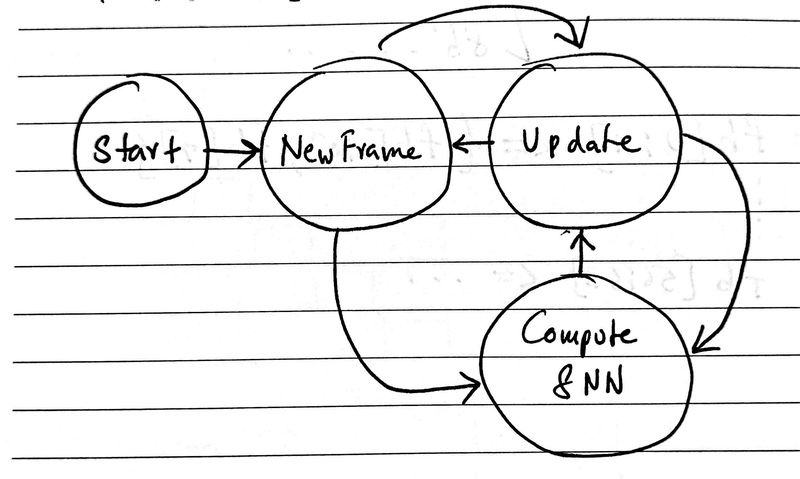Game of Life – State Machine

On start, the state changes to “New Frame”. This initialises the simulation grid and changes state to “Compute 8NN”, which computes the sum of the 8 nearest neighbours of the current pixel. The state then changes to “Update Grid” which keeps switching back and forth with “Compute 8NN” till the whole frame is done, after which state is reset to “New Frame”. Some code snippets of this state machine can be seen below.

// handle state machine
case (curr_state)

sSTART:
begin
// start new frame
curr_state <= sNEW_FRAME;
end

sNEW_FRAME:
begin
// start new frame
if (!cnewframe)
begin
// copy grid
grid_cpy <= grid;

// reset current position
i <= 0;

// change state to compute sum
curr_state <= sCOMPUTE_8NN;
end
end

sUPDATE:
begin

// increment position
i <= i + 1;

// set grid value based on sum
if (grid[i])
begin
if (sum < 2 || sum > 3)
begin
grid[i] <= 0;
end
end
else if (sum == 3)
begin
grid[i] <= 1;
end

// done with frame?
if (i == 63)
begin
// go to new frame
curr_state <= sNEW_FRAME;
end
else
begin
// reset sum
sum <= 0;
// change state to compute
curr_state <= sCOMPUTE_8NN;
end
end

sCOMPUTE_8NN:
begin
// automatic toroidal boundary conditions because of
// index overflow
sum <=  grid_cpy[i-N-1] + grid_cpy[i-N] +
grid_cpy[i-N+1] +
grid_cpy[i-1] + grid_cpy[i+1] +
grid_cpy[i+N-1] + grid_cpy[i+N] +
grid_cpy[i+N+1];

// change state to update
curr_state <= sUPDATE;
end

default:
curr_state <= sSTART;

endcase


Coming from the microcontroller world, writing code for an FPGA in Verilog is a bit weird for me. But it’s also strangely simpler, and one develops a heightened awareness of what’s happening at every tick of the clock, so to speak.

In this pattern, we just want to blink alternate LEDs on the grid. The code for this module is in rain88.v. (Yes, I had fantasies of making it look like rain. It didn’t.)

always @ (posedge clk)
begin
if (!resetn)
begin

// reset counters
refresh_counter <= 0;

// initialise grid
grid[0:7]   <= 8'b01010101;
grid[8:15]  <= 8'b10101010;
grid[16:23] <= 8'b01010101;
grid[24:31] <= 8'b10101010;
grid[32:39] <= 8'b01010101;
grid[40:47] <= 8'b10101010;
grid[48:55] <= 8'b01010101;
grid[56:63] <= 8'b10101010;
end

// update refresh counter
refresh_counter <= refresh_counter + 1;

// update frame
if (!refresh_counter)
begin
grid <= ~grid;
end
end


After initialising a “0101…” pattern, on each clock edge, we just invert the bits to get a blinky grid.

#### Putting it all together

Now that we have our three patterns, all we need is some code to cycle through them. This is done by the module in top.v.

// LED pattern
parameter PAT_LETTERS   = 2'b00;
parameter PAT_CONWAY    = 2'b01;
parameter PAT_RAIN      = 2'b10;
reg [1:0] curr_patt;
...

// assign buffer based on curent pattern
wire [63:0] w_fb = (curr_patt == PAT_LETTERS) ? w_fb_lett :
((curr_patt == PAT_CONWAY) ?
w_fb_conway : w_fb_rain);
...
// letters module
wire [63:0] w_fb_lett;
letters88 let (
.resetn(resetn),
.clk(clk),
.fb(w_fb_lett)
);

// rain module
wire [63:0] w_fb_rain;
rain88 rn(
.resetn(resetn),
.clk(clk),
.fb(w_fb_rain)
);

// Conway's GOL
wire [63:0] w_fb_conway;
conway88 c88 (
.resetn(resetn),
.clk(clk),
.fb(w_fb_conway)
);

// instatiate dot88
dot88 d88(
.resetn(resetn),
.clk(clk),
.fb(w_fb),
.row(row),
.col(col)
);

always @(posedge clk)
begin
// initialise rot
if (!resetn)
begin
counter <= 0;
curr_patt <= PAT_LETTERS;
sw_counter <= 0;
end
else
begin

// increment counters
counter <= counter + 1;
sw_counter <= sw_counter + 1;

// switch pattern
if (!sw_counter)
curr_patt <= curr_patt + 1;

if (!counter)
begin
L2 = ~L2;
end
end



The above shows code snippets for setting up the current pattern and module instantiation. Notice how the wire w_fb, the input to dot88 changes combinatorially based on the current pattern. Every 10 seconds or so, we switch the current pattern which is sent to the dot88.v module we discussed in the beginning.

### Using icestorm tools

For this project, I’ve use the open source icestorm tools from Clifford Wolf, on Linux. These tools are much more convenient to use than the clunky official software put out by Lattice Semiconductor.

To build the project, install icestorm tools, use ‘make‘, and to upload the code, use ‘make sudo-prog‘.

To upload code to iCEBling, we used the Adafruit FT232H board. Here is the pin mapping from FT232H to iCE Bling.

**iCE Bling FT232H
SS D4
SCK D0
MOSI D2
MISO D1
CRESET D7
CDONE D6
GND GND

(Power iCE Bling using coin cell while programming.)

### In Action

You can see iCE Bling in action below:

### Conclusion

So I did it. FPGA earrings, and on time for her birthday. She is thrilled! In retrospect, I should’ve used a shift register. Well, maybe in the next revision.